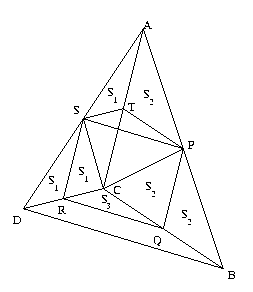#### You may also like### Polycircles

Show that for any triangle it is always possible to construct 3 touching circles with centres at the vertices. Is it possible to construct touching circles centred at the vertices of any polygon?### DOTS Division

Take any pair of two digit numbers x=ab and y=cd where, without loss of generality, ab > cd . Form two 4 digit numbers r=abcd and s=cdab and calculate: {r^2 - s^2} /{x^2 - y^2}.### Loopy

Investigate sequences given by $a_n = \frac{1+a_{n-1}}{a_{n-2}}$ for different choices of the first two terms. Make a conjecture about the behaviour of these sequences. Can you prove your conjecture?

##### Age 14 to 16Challenge Level

Like Quad in Quad (June 1998) the key to this problem is using the fact that, when the midpoints of the sides of a triangle are joined, the join is parallel to the third side and half the length of the third side.

This solution came from Murat from Turkey.Due to the Thales relationship, SP and QR are both parallel to BD, thus to each other. Moreover they are half as long as BD. A similar relationship holds among PQ, RS and AC. Therefore PQRS is a parallelogram.

Let T be the midpoint of AC.

In the above figure, the areas of the triangles marked by the same symbol are obviously equal (look for triangles on equal bases which have the same height). Moreover Area (CST) = S 1 , Area (CPT)= S 2 and Area (TPS)= S 3 . The following equations are obviously true:

 Area (ABCD) = 4( S 1 + S 2 ) Area (PQRS) = ( S 1 + S 2 + S 3 ) + ( S 1 + S 2 - S 3 ) = 2( S 1 + S 2 ) = $\frac {1}{2}$ Area (ABCD)